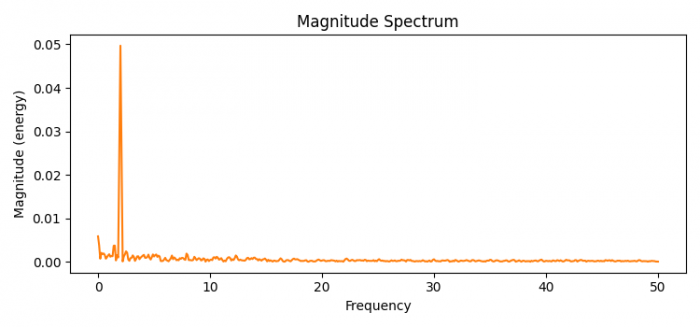# How to plot magnitude spectrum in Matplotlib in Python?

To plot magintude spectrum, we can take the following steps −

• Set the figure size and adjust the padding between and around the subplots.
• Get random seed value.
• Initialize dt for sampling interval and find the sampling frequency.
• Create random data points for t.
• To generate noise, get nse, r, cnse and s using numpy
• Create a figure and a set of subplots, using subplots() method.
• Set the title of the plot.
• Plot the magnitude spectrum.
• To display the figure, use show() method.

## Example

import matplotlib.pyplot as plt
import numpy as np

plt.rcParams["figure.figsize"] = [7.50, 3.50]
plt.rcParams["figure.autolayout"] = True

np.random.seed(0)

dt = 0.01 # sampling interval
Fs = 1 / dt # sampling frequency
t = np.arange(0, 10, dt)

# generate noise:
nse = np.random.randn(len(t))
r = np.exp(-t / 0.05)
cnse = np.convolve(nse, r) * dt
cnse = cnse[:len(t)]
s = 0.1 * np.sin(4 * np.pi * t) + cnse

fig, axs = plt.subplots()

axs.set_title("Magnitude Spectrum")
axs.magnitude_spectrum(s, Fs=Fs, color='C1')

plt.show()

## Output